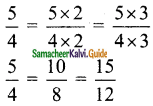Students can download Maths Chapter 3 Ratio and Proportion Ex 3.2 Questions and Answers, Notes, Samacheer Kalvi 6th Maths Guide Pdf helps you to revise the complete Tamilnadu State Board New Syllabus, helps students complete homework assignments and to score high marks in board exams.

## Tamilnadu Samacheer Kalvi 6th Maths Solutions Term 1 Chapter 3 Ratio and Proportion Ex 3.2

Question 1.
Fill in the blanks of the given equivalent ratios.
(i) 3 : 5 = 9 : ……
(ii) 4 : 5 = …… : 10
(iii) 6 : …… = 1 : 2
Solution:
(i) 15
Hint: $$\frac{3}{5}=\frac{3 \times 3}{5 \times 3}=\frac{9}{15}$$
(ii) 8
Hint: $$\frac{4}{5}=\frac{4 \times 2}{5 \times 2}=\frac{8}{10}$$
(iii) 12
Hint: $$\frac{1}{2}=\frac{1 \times 6}{2 \times 6}=\frac{6}{12}$$

Question 2.
Complete the table.Solution:
(i) 1 feet = 12 inches
3 feet = 3 × 12 inches = 36 inches
72 inches = 6 × 12 inches = 6 feet

(ii) 1 week = 7 days
2 weeks = 2 × 7 days = 14 days
63 days = 9 × 7 days = 9 weeksQuestion 3.
Say True or False.
(i) 5 : 7 is equivalent to 21 : 15
(ii) If 40 is divided in the ratio 3 : 2, then the larger part is 24
Solution:
(i) False
Hint: $$\frac{21}{15}=\frac{7}{5}=7: 5$$
(ii) True
Hint: $$\frac{3}{5} \times 40=24$$

Handy LCM of two or more numbers Calculator displays LCM of 365, 838, 862 in a fraction of seconds i.e.

Question 4.
Give two equivalent ratios for each of the following.
(i) 3 : 2
(ii) 1 : 6
(iii) 5 : 4
Solution:
(i) 3 : 23 : 2 = 6 : 4 = 9 : 6

(ii) 1 : 61 : 6 = 2 : 12 = 3 : 18

(iii) 5 : 45 : 4 = 10 : 8 = 15 : 12Question 5.
Which of the two ratios is larger?
(i) 4 : 5 or 8 : 15
(ii) 3 : 4 or 7 : 8
(iii) 1 : 2 or 2 : 1
Solution:
(i) 4 : 5 (or) 8 : 154 : 5 > 8 : 15

(ii) 3 : 4 (or) 7 : 87 : 8 > 3 : 4

(iii) 1 : 2 (or) 2 : 1
1 : 2 = $$\frac{1}{2}$$
2 : 1 = $$\frac{2}{1}$$
= 2
$$\frac{2}{1}$$ > $$\frac{1}{2}$$
2 : 1 > 1 : 2Question 6.
Divide the numbers given below in the required ratio.
(i) 20 in the ratio 3 : 2
(ii) 27 in the ratio 4 : 5
(iii) 40 in the ratio 6 : 14.
Solution:
(i) Ratio = 3 : 2
Sum of the ratio = 3 + 2 = 5
5 parts = 20
1 part = $$\frac{20}{5}$$ = 4
3 parts = 3 × 4 = 12
2 parts = 2 × 4 = 8
20 can be divided in the form as 12, 8.

(ii) Ratio = 4 : 5
Sum of the ratio = 4 + 5 = 9
9 parts = 27
1 part = $$\frac{27}{9}$$ = 3
4 parts = 4 × 3 = 12
5 parts = 5 × 3 =15
27 can be divided in the form as 12, 15.

(iii) 40 in the ratio 6 : 14
Ratio = 6 : 14
Sum of the ratio = 6 + 14 = 20
20 parts = 40
1 part = $$\frac{40}{20}$$ = 2
6 parts = 2 × 6 = 12
14 parts = 2 × 14 = 28
40 can be divided in the form as 12, 28.Question 7.
In a family, the amount spent in a month for buying Provisions and Vegetables are in the ratio 3 : 2. If the allotted amount is Rs 4000, then what will be the amount spent for
(i) Provisions and
(ii) Vegetables?
Solution:
Allotted amount = Rs 4000
Ratio = 3 : 2
Sum of the ratio = 3 + 2 = 5
5 parts = Rs 4000
1 part = Rs $$\frac{4000}{5}$$ = Rs 800
Provisions : Vegetables = 3 : 2
3 parts = 3 × Rs 800 = Rs 2400
2 parts = 2 × Rs 800 = Rs 1600
Amount spent for provisions = Rs 2400
Amount spent for vegetables = Rs 1600Question 8.
A line segment 63 cm long is to be divided into two parts in the ratio 3 : 4. Find the length of each part.
Solution:
Total length = 63 cm Ratio = 3 : 4
Sum of the ratio = 3 + 4 = 7
7 parts = 63 cm
1 part = $$\frac{63}{7}$$ = 9 cm
3 parts = 3 × 9 cm = 27 cm
4 parts = 4 × 9 cm = 36 cm
∴ 63 cm can be divided into the parts as 27 cm and 36 cm.

Objective Type Questions

Question 9.
If 2 : 3 and 4 : …… are equivalent ratios, then the missing term is
(a) 6
(b) 2
(c) 4
(d) 3
Solution:
(a) 6Question 10.
An equivalent ratio of 4 : 7 is
(a) 1 : 3
(b) 8 : 15
(c) 14 : 8
(d) 12 : 21
Solution:
(d) 12 : 21

Question 11.
Which is not an equivalent ratio of $$\frac{16}{24}$$?
(a) $$\frac{6}{9}$$
(b) $$\frac{12}{18}$$
(c) $$\frac{10}{15}$$
(d) $$\frac{20}{28}$$
Solution:
(d) $$\frac{20}{28}$$Question 12.
If Rs 1600 is divided
(a) Rs 480
(b) Rs 800
(c) Rs 1000
(d) Rs 200
Solution:
(c) Rs 1000